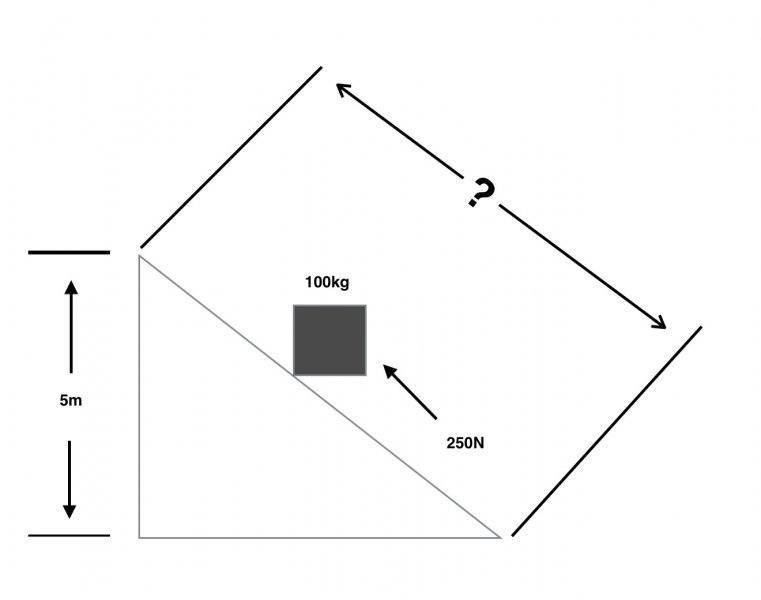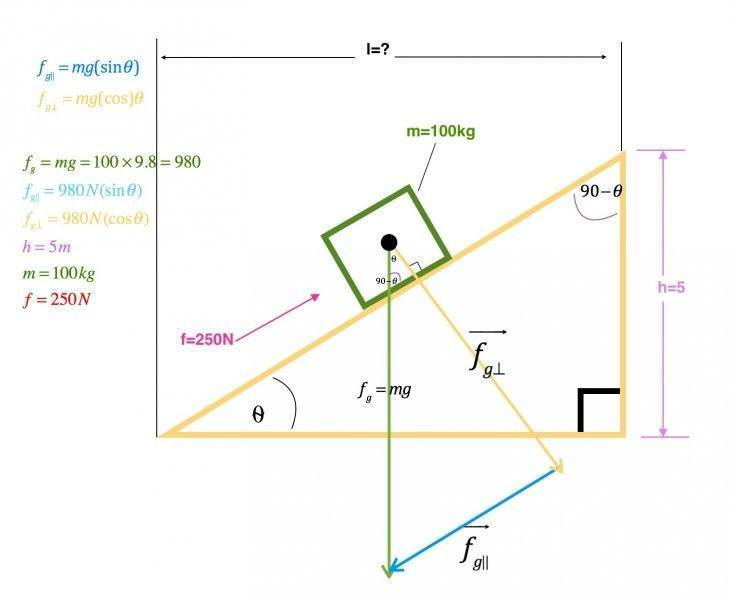# Simple incline problem has me confused

## Homework Statement

1. An inclined plane is used to raise a 100 kg box to a height of 5 m. How long must the inclined plane be if a force of 250 N is used to push the box up the inclined plane?

## Homework Equations

i have search and searched all over google to try to figure this out.. I am not sure if I'm over thinking it or just confused myself even more. I found one equation that I think is correct, but as i was looking at other examples I tried to use this formula and it never worked out like I thought it should. Also, I am always finding examples that have an angle of inclination.. But the formula I think should work is.

$$\frac{f}{w}=\frac{h}{l}$$

## The Attempt at a Solution

$$\frac{250}{100}=\frac{5}{l}$$
l=2???

cheers
keith

NascentOxygen
Staff Emeritus
Hi keithcuda. http://img96.imageshack.us/img96/5725/red5e5etimes5e5e45e5e25.gif [Broken]

You won't be able to avoid involving either sinθ or cosθ in your calculations.
Can you attach a pic showing the relevant diagram you have drawn for this problem?

Last edited by a moderator:
You could draw a free body diagram to see what forces are opposing the motion of the box on an incline. For there to be an upward motion, that or those force(s) must be less than the upward force.

i can try to draw pic and post it...

That is where i am confused.. the question isn't giving me an angle of the incline.. so how do i include sinθ or cosθ?

Maybe one of the opposing forces is related to the angle ##\theta## of the incline.

NascentOxygen
Staff Emeritus
θ
i can try to draw pic and post it...

That is where i am confused.. the question isn't giving me an angle of the incline
So call the slope θ, or x or something.

Hi keithcuda. http://img96.imageshack.us/img96/5725/red5e5etimes5e5e45e5e25.gif [Broken]

You won't be able to avoid involving either sinθ or cosθ in your calculations.
Can you attach a pic showing the relevant diagram you have drawn for this problem?#### Attachments

Last edited by a moderator:
NascentOxygen
Staff Emeritus
OK. Next show a vector representing the force of gravity on that box. What magnitude would this force have? And its units?

Have you given the slope a name, i.e., a letter?

OK, so
OK. Next show a vector representing the force of gravity on that box. What magnitude would this force have? And its units?

Have you given the slope a name, i.e., a letter?

Ok, so I did some more googling...
I found this, Fp=w(h/l)
Fp=250N
w=mg=100*9.8
h=5
l=slope
250=980(5/l)
l=19.6m ?

let me know what I am doing wrong or missing and as soon as i leave work and get home I'll work on it some more

cheers

NascentOxygen
Staff Emeritus
Once you have answered the questions in post #8 ....

gravitational force on the box can be resolved into an equivalent pair of forces at right-angles to each other: one force acting parallel to the plane, and the second force acting perpendicular to the plane

Forces are vectors, so to add them you must add them as vectors. Draw this "triangle of forces" to show these two perpendicular forces summing to equal the gravitational force on the box. Use trigonometry to calculate the magnitudes of the unknown sides of this triangle.

Alright, I believe I know what you are talking about... But, I'm just getting home from my power plant after working a 17 hour shift. Once I get some sleep, I'll work on post #8 and #11.

NascentOxygen
Staff Emeritus
If you need help with the vector diagram, search on google images for "block on inclined plane". You'll get hundreds. :w

http://thumbnails112.imagebam.com/37333/0363e9373324851.jpg [Broken]

Last edited by a moderator:
If you need help with the vector diagram, search on google images for "block on inclined plane". You'll get hundreds. :w

http://thumbnails112.imagebam.com/37333/0363e9373324851.jpg [Broken]
Thank you, I'm actually working on it right now :)

Last edited by a moderator:
OK, so here is what I put together so far...NascentOxygen
Staff Emeritus
So far so good ....

So far so good ....
I guess this is where I am really stuck. Without a given angel for theta, how to I proceed with my problem?

g assumed at 9.81 m/s/s
Choose any incline angle (and therefore incline length) but dont exceed 14.764 ° incline though, as you wont have any force left to accelerate the mass up the incline.
The problem is, if the force is kept constant, at the top of the slope, the mass will still be moving (and accelerating).

So, you accelerate the mass so far up the slope then remove the (250 N) force and allow it to decelerate under gravity, to come to a rest at the top of the slope.
Ive ran a few examples through, and found that :
Regardless of the incline length, the length you apply the force over turns out to be the same.
The key is that you put the work in ( force * distance) which is equal to the final PE (m*g*h)
So :
250 * d = m * g * h
You may do the rest.

NascentOxygen
Staff Emeritus
I guess this is where I am really stuck. Without a given angel for theta, how to I proceed with my problem?
The problem includes a force 250N. Of the expressions you have derived, what can you equate to 250N?

g assumed at 9.81 m/s/s
Choose any incline angle (and therefore incline length) but dont exceed 14.764 ° incline though, as you wont have any force left to accelerate the mass up the incline.
The problem is, if the force is kept constant, at the top of the slope, the mass will still be moving (and accelerating).

So, you accelerate the mass so far up the slope then remove the (250 N) force and allow it to decelerate under gravity, to come to a rest at the top of the slope.
Ive ran a few examples through, and found that :
Regardless of the incline length, the length you apply the force over turns out to be the same.
The key is that you put the work in ( force * distance) which is equal to the final PE (m*g*h)
So :
250 * d = m * g * h
You may do the rest.
so, 250*d=100*9.8*5
d=4900/250
d=19.6

The problem includes a force 250N. Of the expressions you have derived, what can you equate to 250N?
I am sorry, but I'm not sure I understand what you are asking :(

NascentOxygen
Staff Emeritus
I am sorry, but I'm not sure I understand what you are asking :(
On your figure you show the pink 250N acting up the slope. You equate this to the blue component of weight acting in the exact opposite direction. You have the equation for that, you called it fg∥.

Thats right, what you find is that if you remove the force at that point, it will decelerate to a stop exactly at the top of the slope.
This d applies regardless of which incline angle you choose ( up to 14.764 °).

Dean Barry

On your figure you show the pink 250N acting up the slope. You equate this to the blue component of weight acting in the exact opposite direction. You have the equation for that, you called it fg∥.
ok so, 250=980(sinθ)
sin(θ)=250/980
θ=sin-1(250/980)
θ=14.77

so if θ=14.77°
then, sin(14.8)=5/h
h=5/sin(14.8)
h=19.57m

NascentOxygen
Staff Emeritus
Looks right. Note: the length of the slope is not the "l" you show on your figure.

In 99% of the problems of this type you are required to find the slope or θ. In today's problem you don't specifically have to. Going back to your figure, you could have looked at similar triangles: the slope triangle, and the weight triangle. This leads to the equating of ratios (which may be what you copied from something on the net way back near the start).

It would be worthwhile making sure you can see those similar triangles, before leaving this problem.

Last edited:
NascentOxygen
Staff Emeritus
I guess this is where I am really stuck. Without a given angel for ....
Fingers crossed that you'll have that angel on your shoulder during exams!http://thumbnails112.imagebam.com/37333/0363e9373324851.jpg [Broken]

Last edited by a moderator:
Fingers crossed that you'll have that angel on your shoulder during exams!http://thumbnails112.imagebam.com/37333/0363e9373324851.jpg [Broken]
haha, good catch ;)

I tend to type too fast and not proofread enough...
Thank you for your help. I appreciate it!
Happy Holidays
Cheers

Last edited by a moderator:
NascentOxygen
Staff Emeritus
The key is that you put the work in ( force * distance) which is equal to the final PE (m*g*h)
So :
250 * d = m * g * h
You may do the rest.
That's an interesting alternative way to work this problem. Perfectly valid, and a good way to confirm ones understanding of kinetics. Well done.

•keithcuda
Heres the excel check sheet (tidied up version) for proof.
The tan cells are inputs (vary if you wish).
Dont exceed the maximum slope angle (Am)
(ive used 10° as an example , alter as you wish)
The two blue cells match regardless of the slope value.
Regards.
Dean

#### Attachments

• jotter.xlsx
11.1 KB · Views: 165
ps work down the sheet from the top, confirm the max allowable incline angle, then input the incline angle (A)

Thats awesome! Thanks a lot..